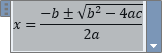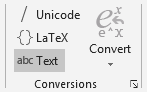﻿ How to change the font of equation formula in Word ? | AnswerTabs

# How to change the font of equation formula in Word ?

Hello, I would like to change the font of equation in Word document, but for some reason, when I choose the required font, it is not changing and there is still Cambria math applied. How can I change the font of created equation formula for example to Arial or Times New Roman ?
Hi,

The change the font of equation in Word, you have to:

Select the equation:Click on Design and in Conversions section select abc Text:Then you can change the font of equation according to your needs.
Related questions